﻿ 航行体出筒过程中弹翼横向偏移研究
 舰船科学技术2022, Vol. 44Issue (19): 178-183    DOI: 10.3404/j.issn.1672-7649.2022.19.037PDF

Lateral displacement of wing on process launching submersible tactical vehicle
CHENG Dong, LU BingJu, ZHU ZhuThe 713 Research Institute of CSSC, Zhengzhou 450015, China; Henan Key Laboratory of Underwater Intelligence Equipment, Zhengzhou 450015, China
Abstract: The deform model and lateral dynamic method and the load calculation method has been established for the adaptation methods of seal-pad in vertical launching submarine-to-air vehicle, and was applied to simulate the process of the vehicle with wings. The influence of the submarine velocity and the rigidity of pad on the lateral displacement of wing and load of the dangerous face and the outlet attitude in vertical launching submarine-to-air vehicle were analyzed. The result shows that on the place of the seal, the lateral displacement of wing relate to the condition of launch as well as the frequency of oscillation. The result has guidance significance to vertical launching submarine-to-air vehicle or other tactical vehicle and design the adapter.
Key words: vehicle     vertical sub-launch     lateral dynamic     seal-pad     lateral displacement
0 引　言

1 模　型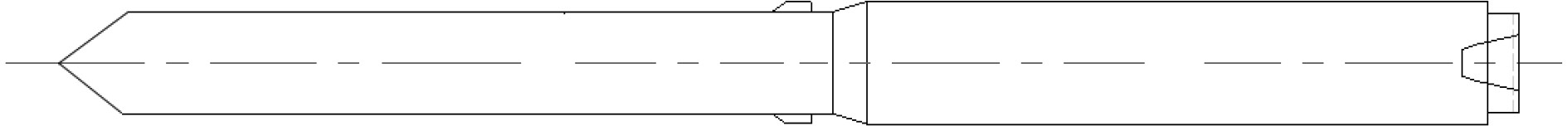图 1 航行体外形示意图 Fig. 1 Shape of the vehicle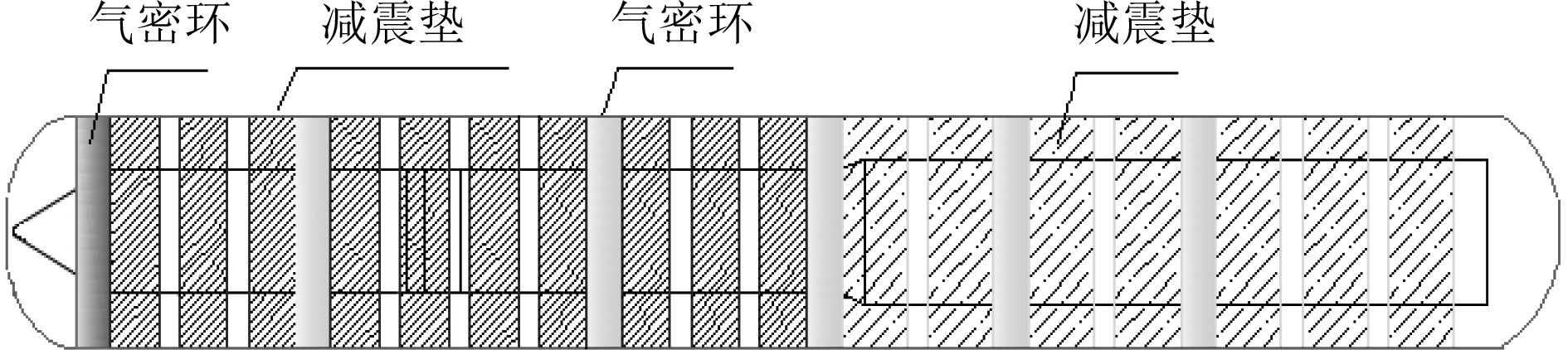图 2 弹筒匹配示意图 Fig. 2 Matching of the vehicle and tube

2 减震垫变形模型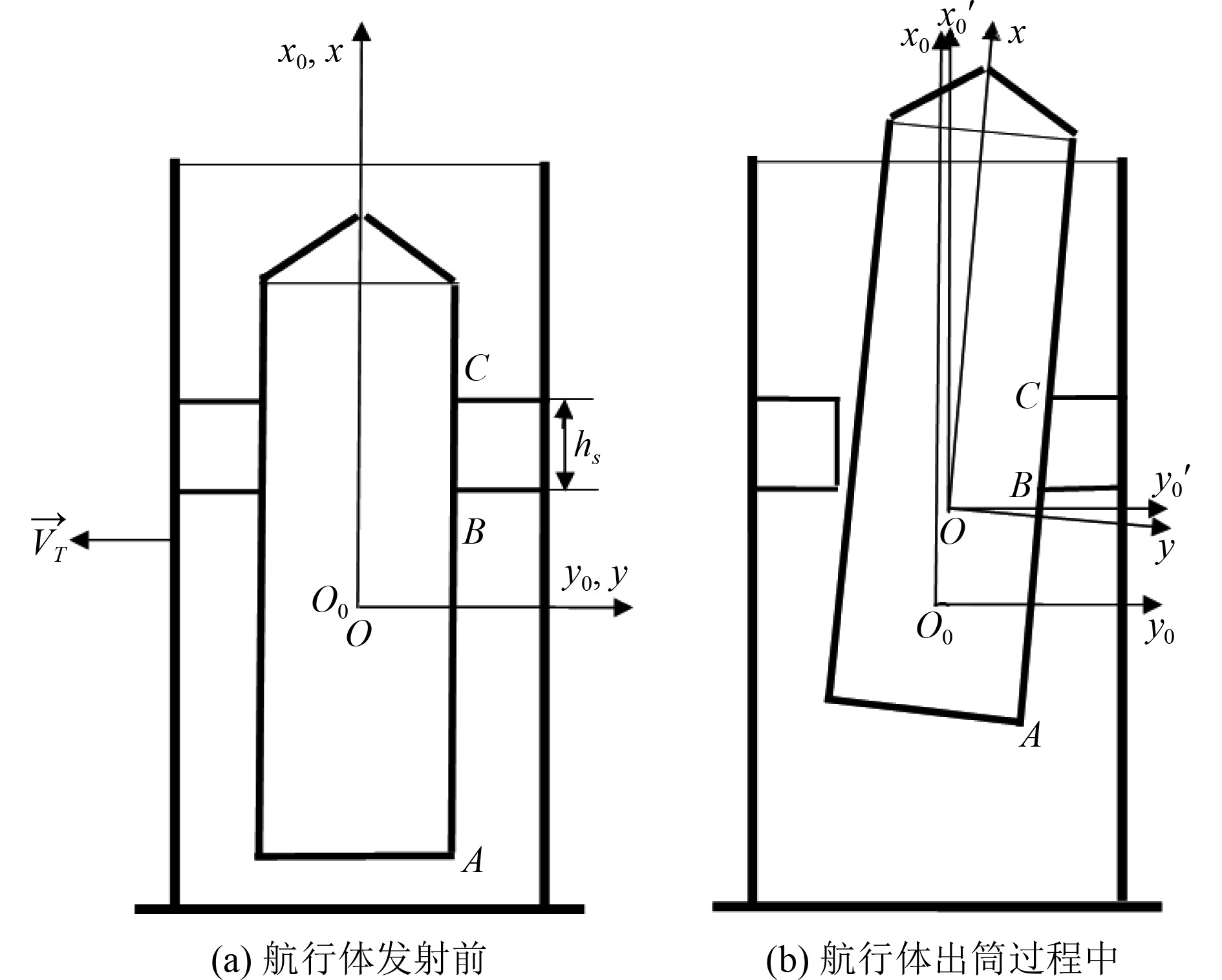图 3 减震垫变形示意图 Fig. 3 Pad deform

1) 如果 ${x_{0A}} \leqslant {x_{0B}}$ ，减震垫完整作用于航行体；

2) 如果 ${x_{0B}} < {x_{0A}} < {x_{0C}}$ ，减震垫部分作用于航行体；

3) 如果 ${x_{0A}} > {x_{0C}}$ ，减震垫完全脱离了航行体。

 ${F_y} = {k_1}\int\nolimits_C^B {\Delta {d_M}{\rm{d}}{h_{SM}}}。$

3 数值计算方法

 ${v_x} = {v_x}(t) ，$
 $\left( {m + {\lambda _{22}}} \right)\frac{{{\rm{d}}{v_y}}}{{{\rm{d}}t}} + {\lambda _{26}}\frac{{{\rm{d}}{\omega _z}}}{{{\rm{d}}t}} + m{v_x}{\omega _z} = Y + {Y_S}，$
 $\left( {{J_z} + {\lambda _{66}}} \right)\frac{{{\rm{d}}{\omega _z}}}{{{\rm{d}}t}} + {\lambda _{26}}\frac{{{\rm{d}}{v_y}}}{{{\rm{d}}t}} = {M_z} + {M_{zS}} ，$
 $\frac{{{\rm{d}}\theta }}{{{\rm{d}}t}} = {\omega _z}，$
 $\frac{{{\rm{d}}{x_0}}}{{{\rm{d}}t}} = {v_x}\cos \theta - {v_y}\sin \theta，$
 $\frac{{{\rm{d}}{y_0}}}{{{\rm{d}}t}} = {v_x}\sin \theta + {v_y}\cos \theta。$

1）初值

2）运动方程组求解

a) $t = t{}_1 + \Delta t$

① 求解运动方程组，计算航行体运动；

②根据航行体横向位移与倾角，计算各减震垫及气密环形变；

③根据变形计算各减震垫及气密环作用力；

④以新的减震垫及气密环作用力计算航行体运动，进行代计算，直至航行体的横向运动参数与减震垫及气密环的变形量趋于一致，停止迭代，转入下一时间步长计算。

b) $t = t{}_1 + \Delta t + \Delta t$

……

c) 直至完全出筒

4 仿真结果及分析 4.1 发射深度影响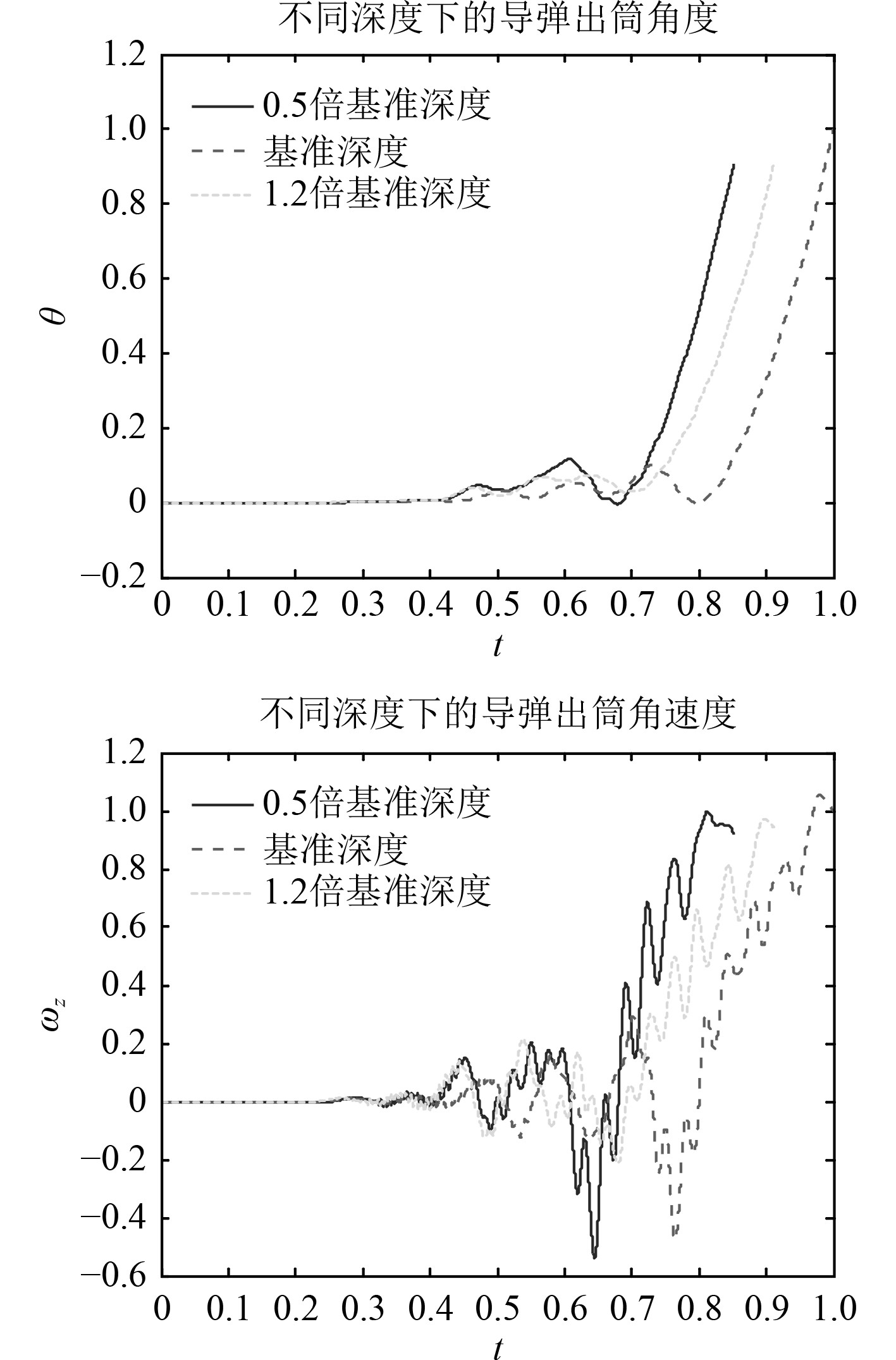图 4 不同深度下的出筒姿态 Fig. 4 Eject attitude with different deep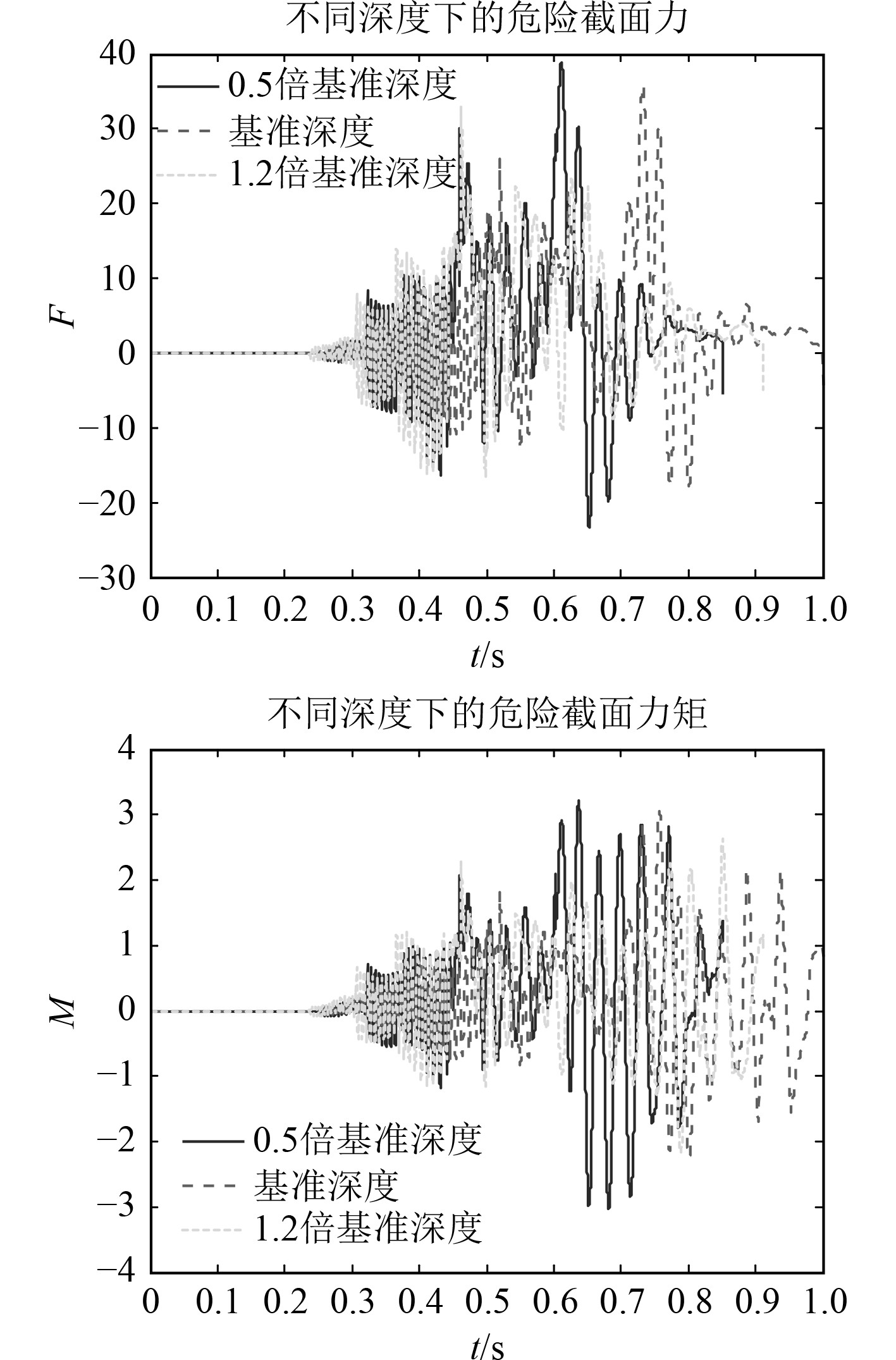图 5 不同深度下的危险截面载荷 Fig. 5 Load of dangerous face with different deep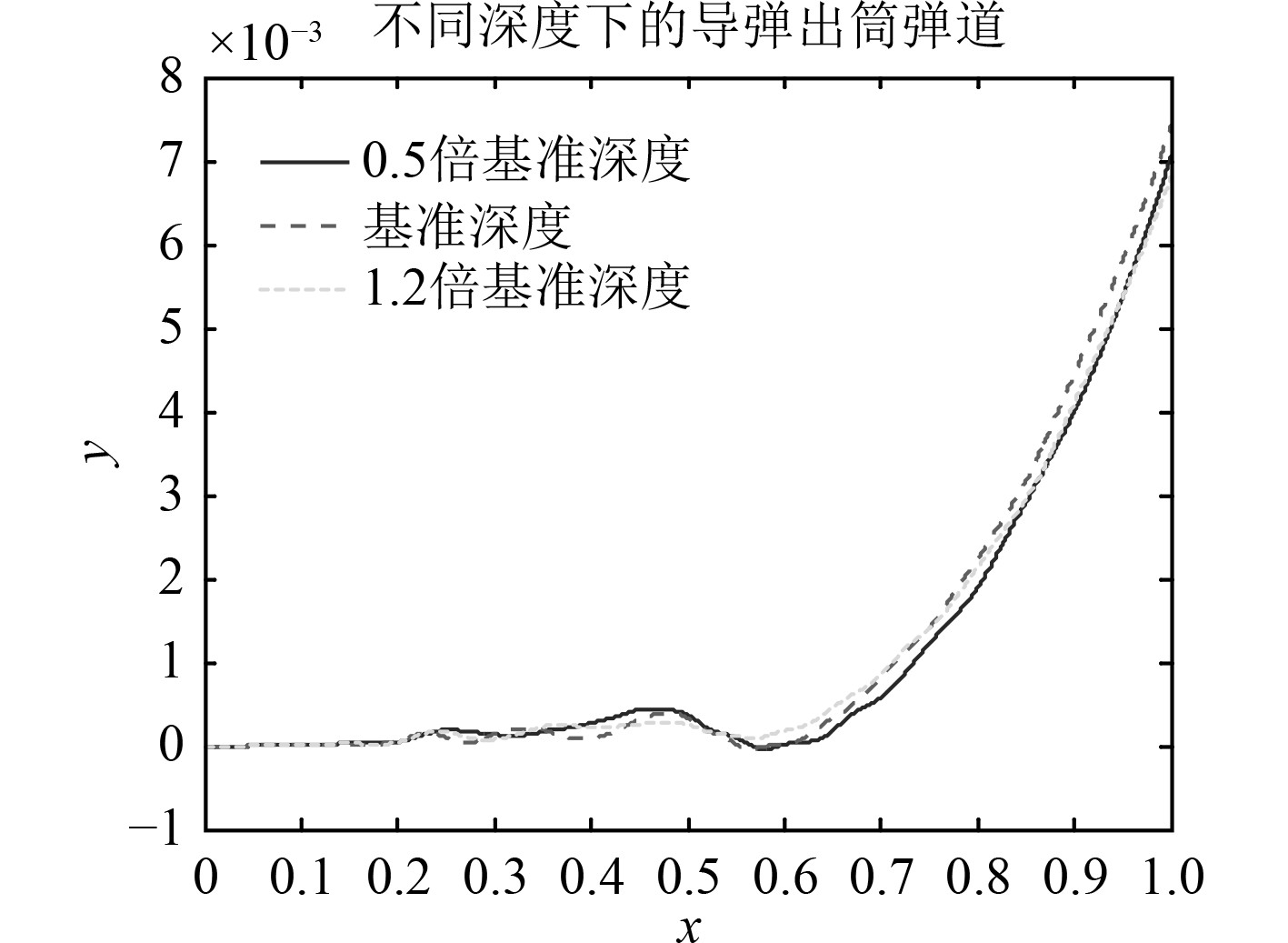图 6 不同深度下的出筒弹道 Fig. 6 Trajectory with different deep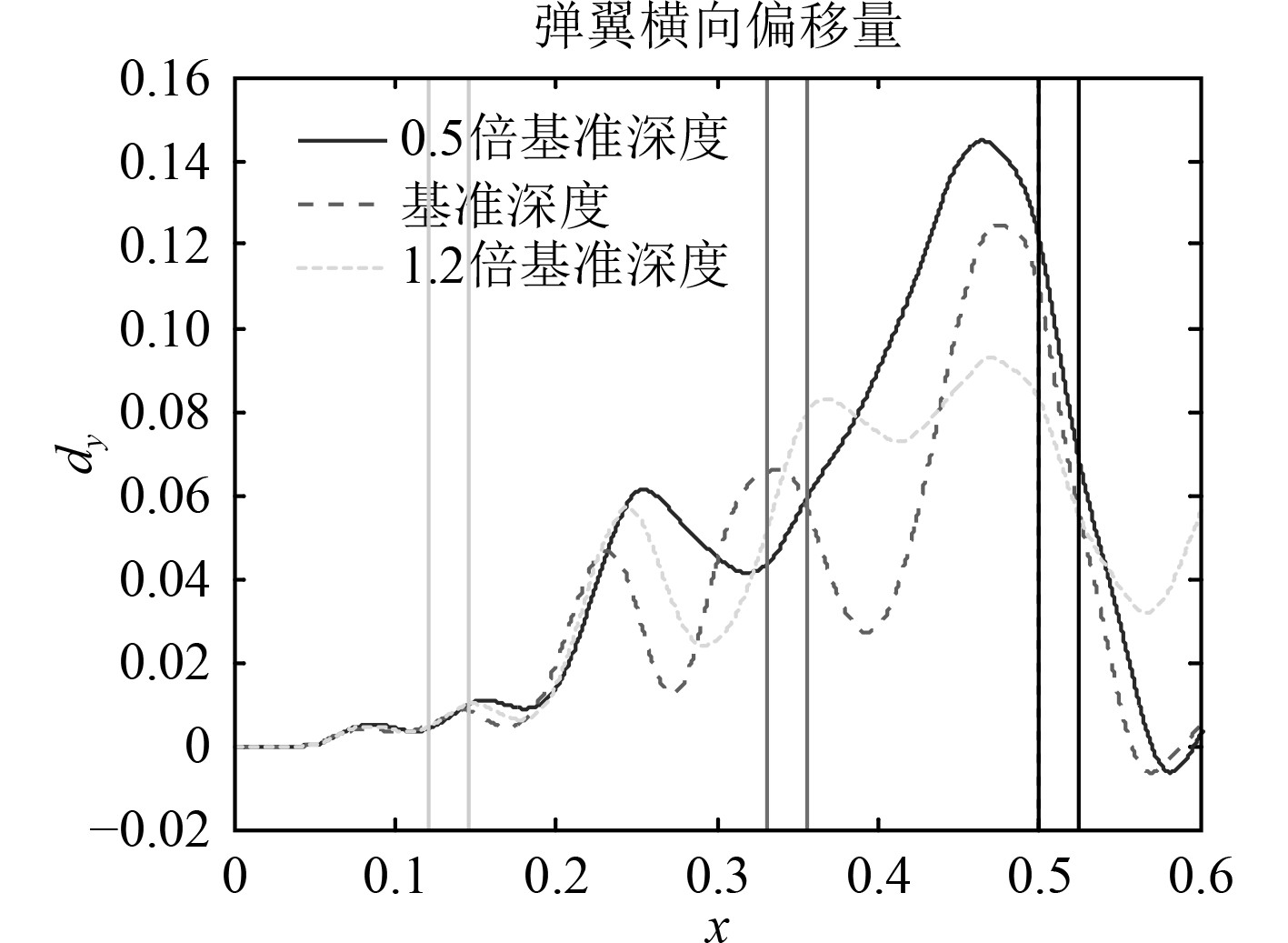图 7 不同深度下的弹翼横移与气密环相对位置 Fig. 7 Wing displacement and seal with different deep表 1 不同发射深度下的结果 Tab.1 result with different deep
4.2 航速影响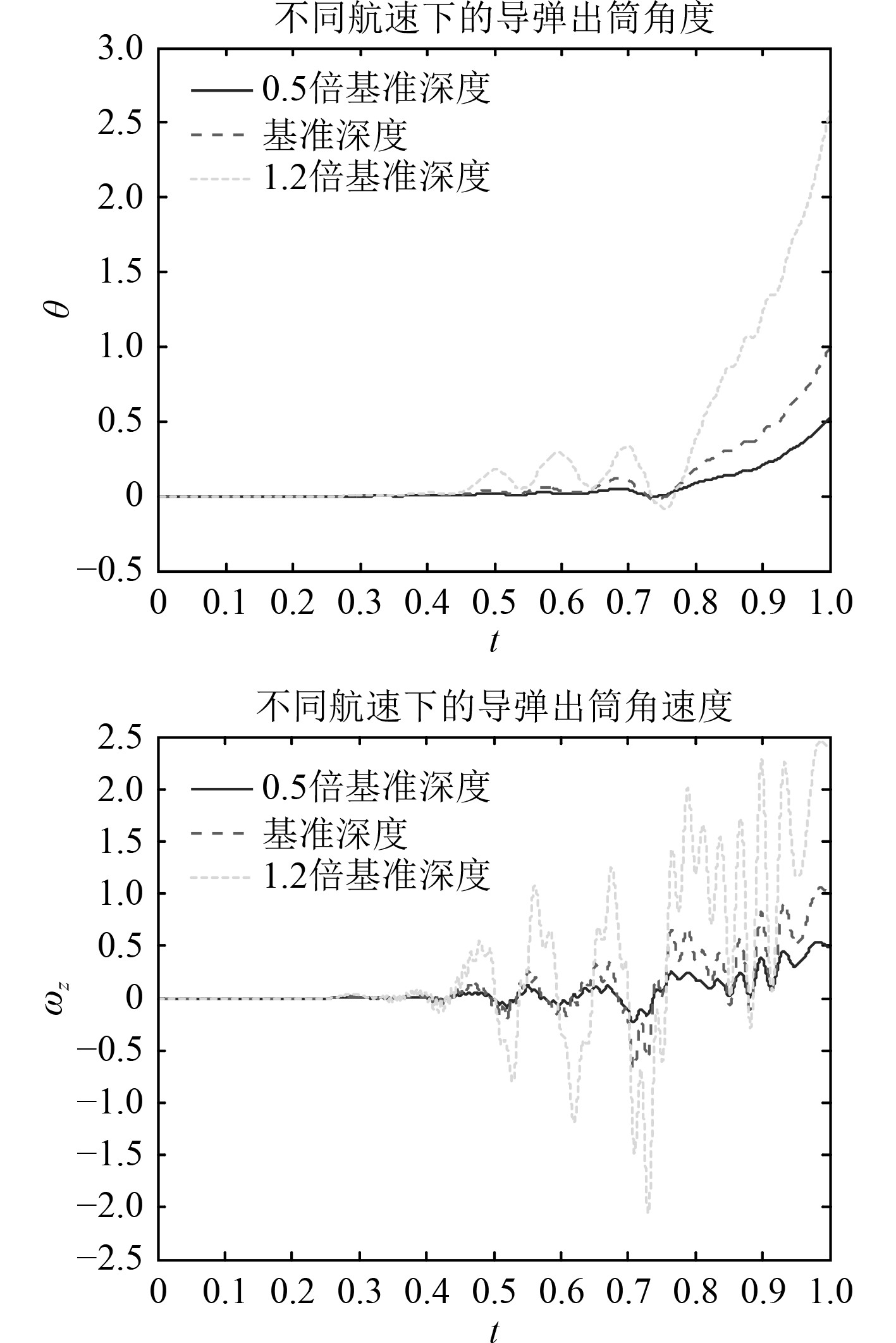图 8 不同航速下的出筒姿态 Fig. 8 Eject attitude with different velocity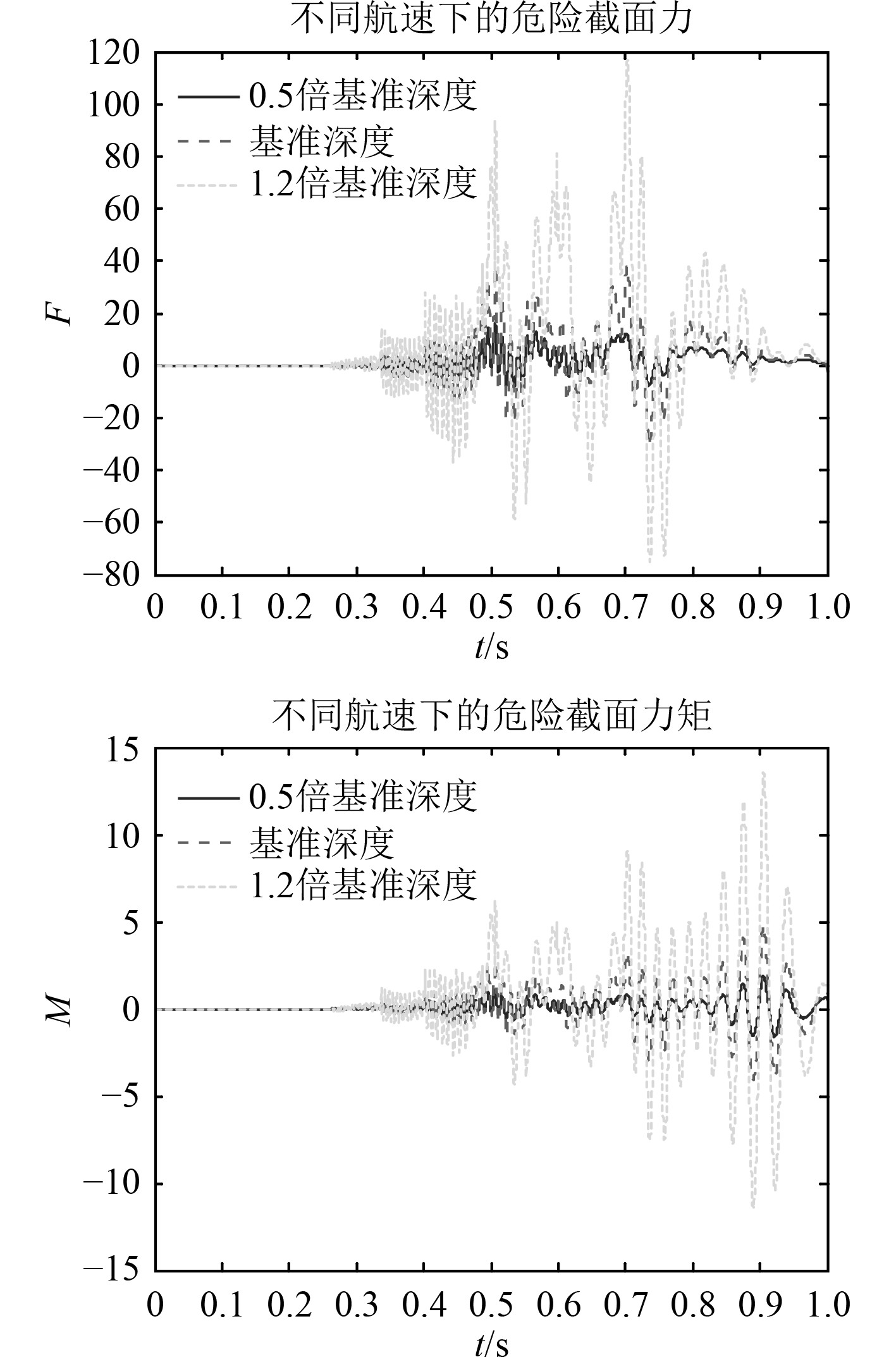图 9 不同航速下的危险截面载荷 Fig. 9 Load of dangerous face with different velocity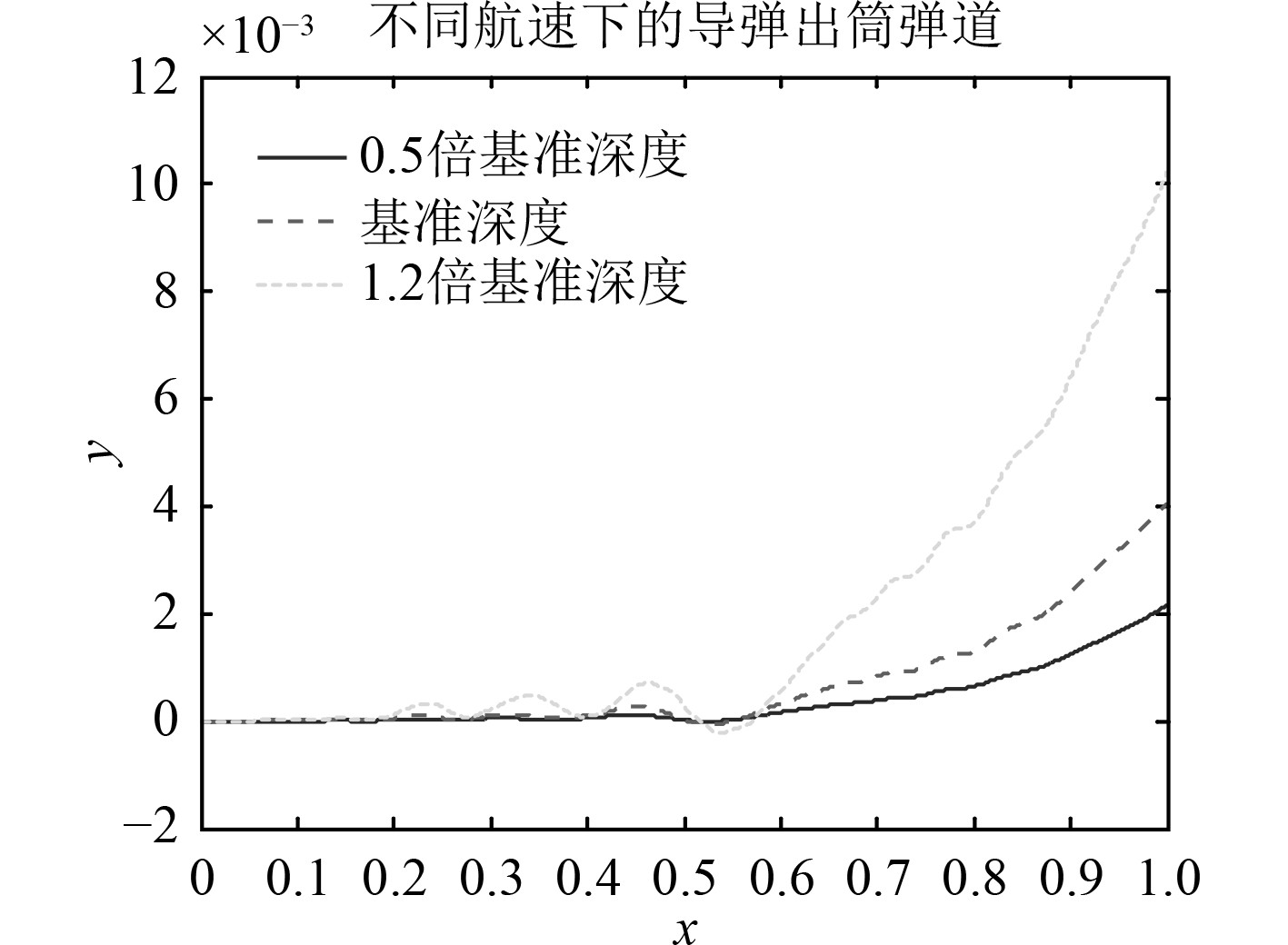图 10 不同航速下的出筒弹道 Fig. 10 Trajectory with different velocity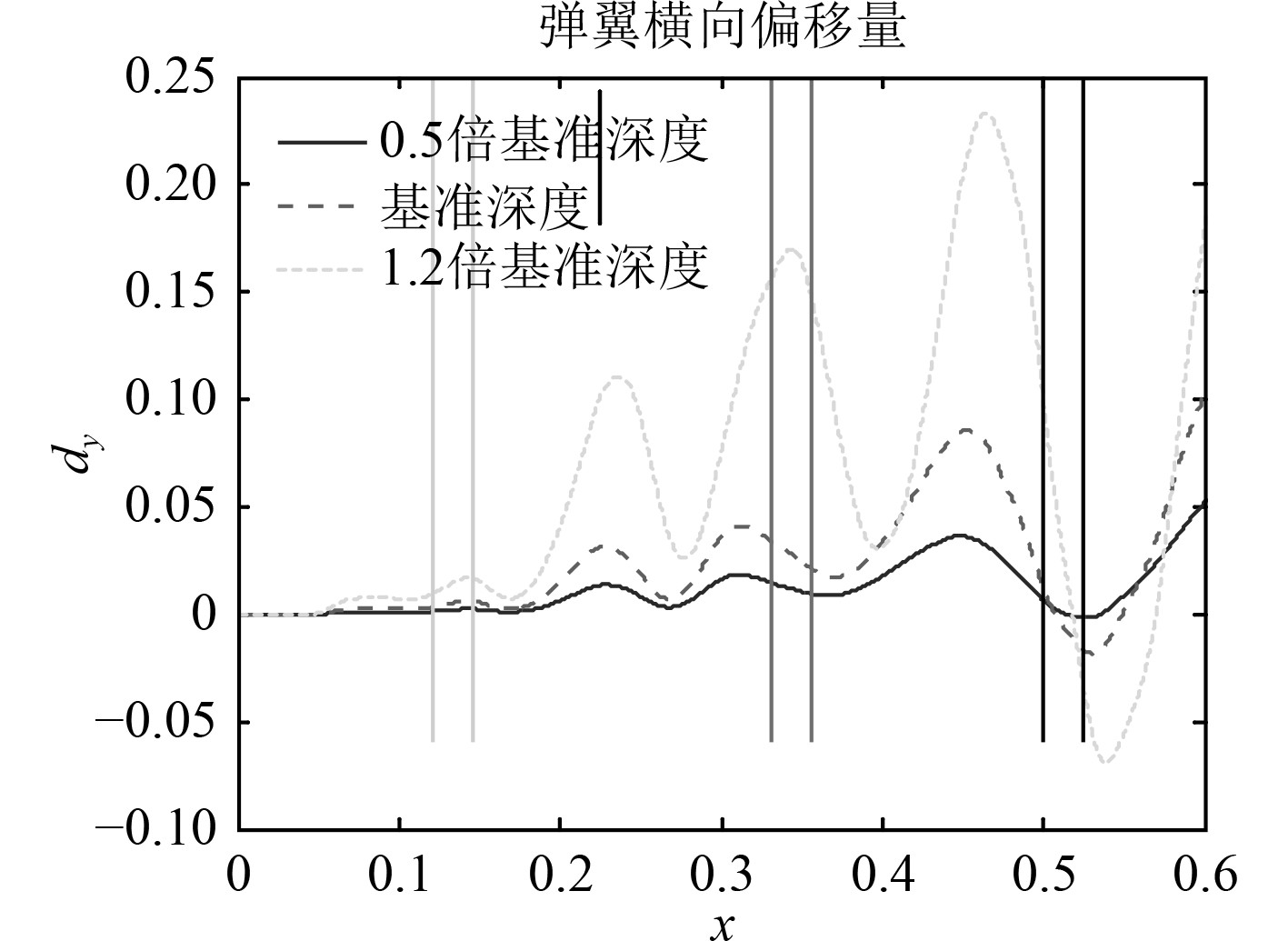图 11 不同航速下的弹翼横移与气密环相对位置 Fig. 11 Wing displacement and seal with different velocity表 2 不同发射航速下的结果 Tab.2 result with different velocity
4.3 减震垫刚度影响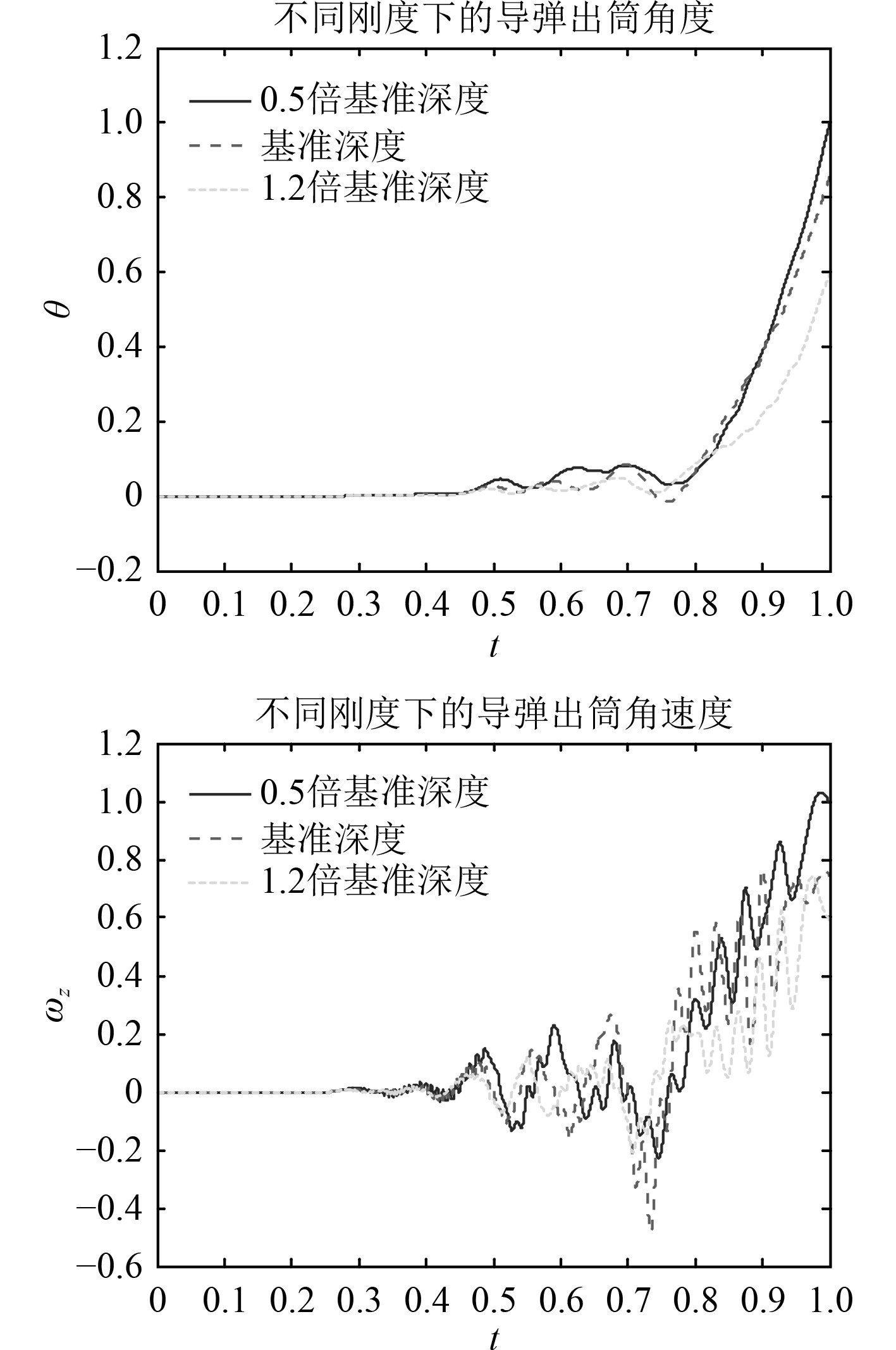图 12 不同刚度下的出筒姿态 Fig. 12 Eject attitude with different stiffness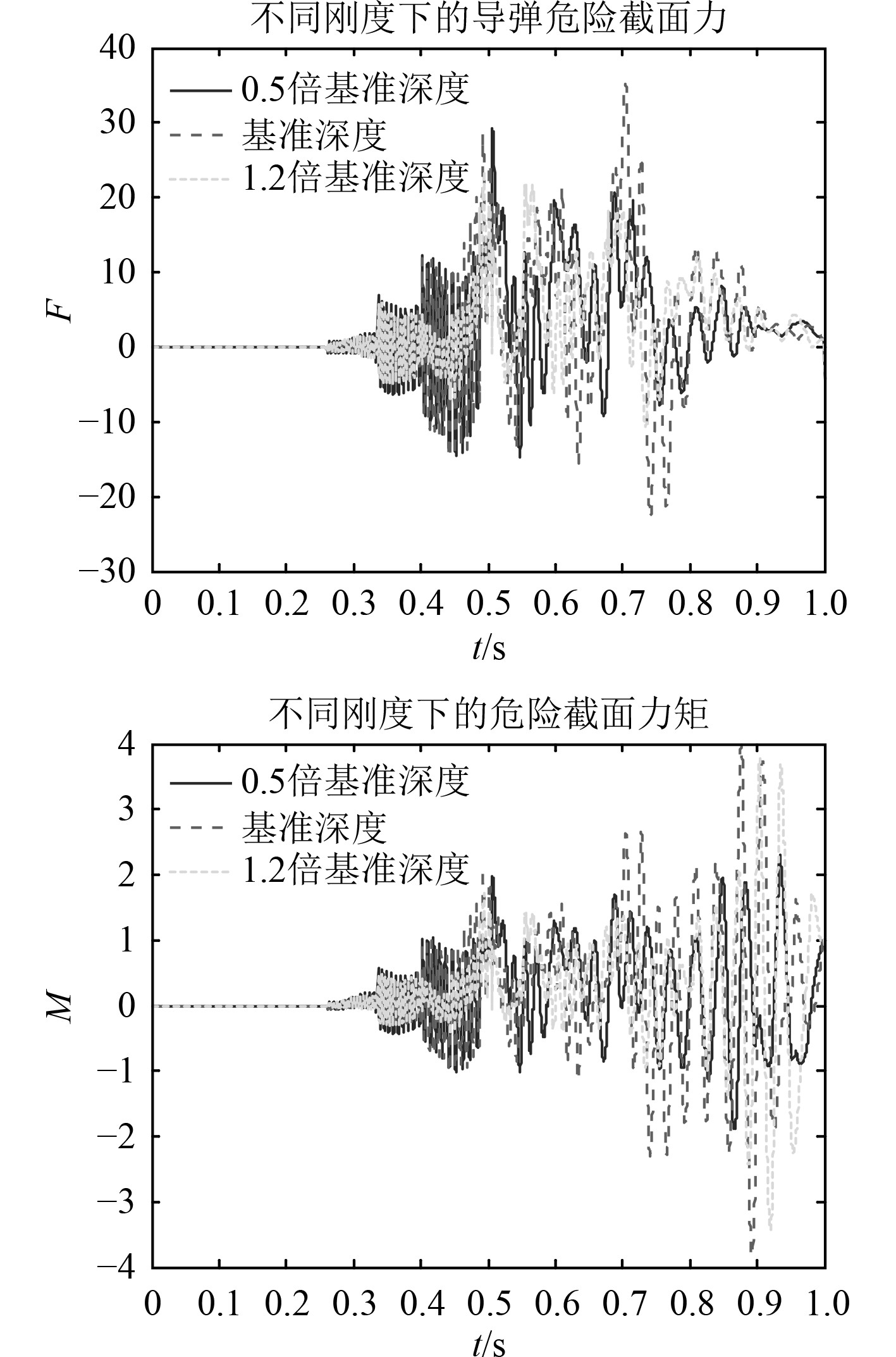图 13 不同刚度下的危险截面载荷 Fig. 13 Load of dangerous face with different stiffness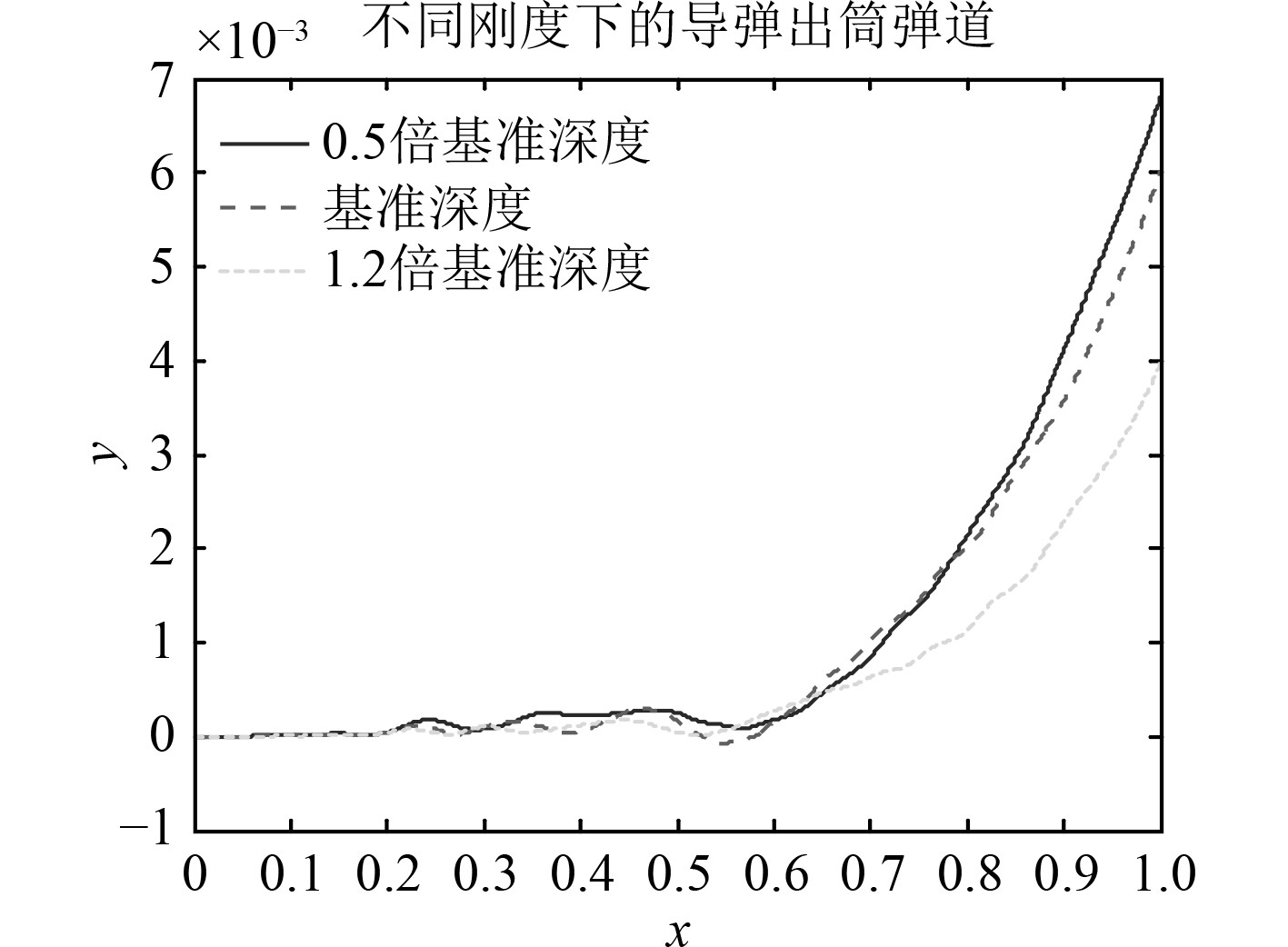图 14 不同刚度下的出筒弹道 Fig. 14 Trajectory with different stiffness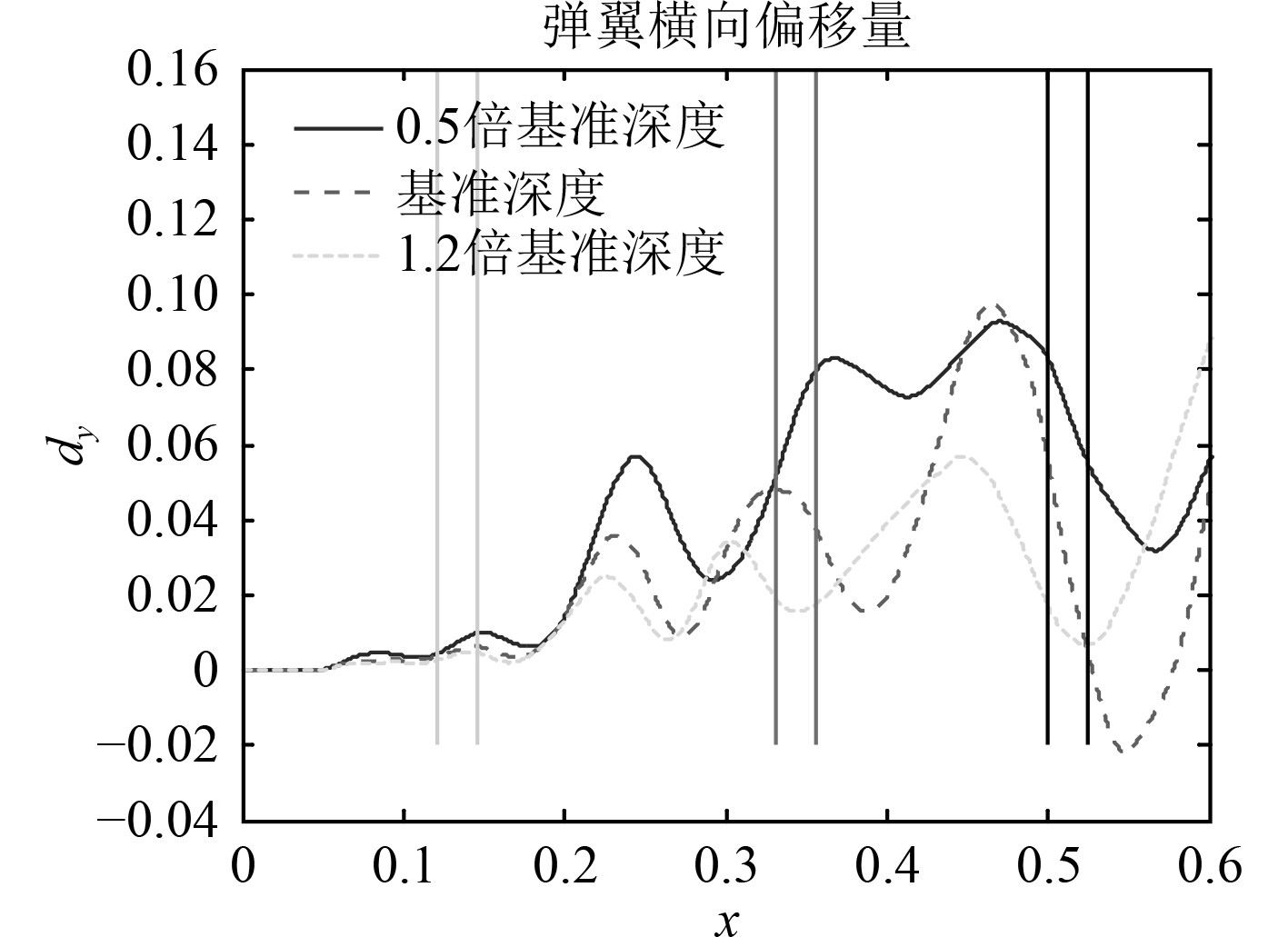图 15 不同刚度下的弹翼横移与气密环相对位置 Fig. 15 Wing displacement and seal with different stiffness表 3 不同减震垫刚度下的结果 Tab.3 result with different stiffness
5 结　语

  尚书聪, 孙建中. 两种弹筒适配方式对航行体出筒姿态的影响[J] 弹道学报, 2012, 24(2): 96-100  赵世平, 蔡体敏. 横向流对潜艇垂直发射航行体的影响[J]. 船舶力学, 2006, 4(10): 33-37  尚书聪, 孙建中, 秦丽萍. 潜载航行体水下发射出筒横向动力学特性研究[J]振动与冲击, 2012, 31(23): 84-86.  朱珠, 卢丙举. 潜空航行体垂直发射出筒横向载荷特性[J]应用力学学报, 2018, 35(3): 452-457.  卢丙举, 朱珠. 潜空航行体垂直发射出筒适配器受载变形仿真研究[J]舰船科学技术, 2017, 39(5): 139-142.